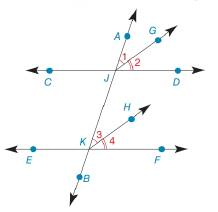Chapter 2.3, Problem 20E### Elementary Geometry for College St...

6th Edition
Daniel C. Alexander + 1 other
ISBN: 9781285195698

#### Solutions

Chapter
Section### Elementary Geometry for College St...

6th Edition
Daniel C. Alexander + 1 other
ISBN: 9781285195698
Textbook Problem
3 views

# In Exercise 19 to 22 complete the proof. Given: ∠ 1 ≅ ∠ 3 ∠ 2 ≅ ∠ 4 Prove: C D ↔ ∥ E F ↔To determine

To find:

The complete proof for the given statement.

Explanation

Given:

The given statement is,

13 and 24

Figure (1)

Theorem:

(1). Congruent angles are of same measure.

(2). If two lines are cut by a transversal so that two corresponding angles are congruent, then these lines are parallel.

Approach:

The given statement is,

13 and 24.

So,

m1=m3m2=m4m(1+2)=m(3+4)mAJD=mJKF

The above equation implies,

AJDJKF

AJD and JKF are two corresponding angles which are congruent to each other.

Thus, CDEF.

The complete proof for the given statement is shown in the following table,

 Proof Statements Reasons 1. ∠1≅∠3 and ∠2≅∠4 1. Given 2

### Still sussing out bartleby?

Check out a sample textbook solution.

See a sample solution

#### The Solution to Your Study Problems

Bartleby provides explanations to thousands of textbook problems written by our experts, many with advanced degrees!

Get Started

#### Find more solutions based on key concepts